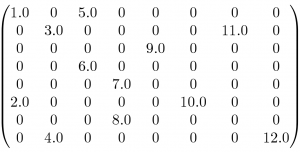8Sep/11Off

## Sparse 101: Matrix Formats

by Dan

With the release of the CULA Sparse beta, we thought it would be useful to present an introduction to sparse matrix formats. Traditionally, a matrix is considered sparse when the number of non-zero elements is significantly less than the number of zero elements. When represented in a program, sparse matrices, unlike the dense matrices used in CULA’s LAPACK functions, are a logical, compressed representation of a matrix. Whereas a dense matrix represents all elements of a matrix, including zeroes, a sparse matrix will represent only non-zero elements. An immediate benefit of this approach is that algorithmic speed improvements can be made by disregarding the zero elements for many operations. An arguably more important benefit (and a focus of this article) is that a representation that stores only non-zero elements allows the total memory used by a sparse matrix to be significantly less than it would be if it were stored densely.Consider an 8x8 matrix, shown to the right. In this matrix, only 12 of the 64 entries (18%) are populated. If we were to adopt a sparse storage format for this matrix, we could reduce the storage by ~60%, from 512 bytes down to 192 with a compressed format.

The simplest compressed format, coordinate (COO), represents a matrix by its non-zero values and an index at which each non-zero is located. For example, in the example matrix, the value 3.0 is located at (2,2) using 1-based indexing. These indices do add to the storage cost for the matrix, but because the number of non-zeros is small, there is a net gain when compared with a dense representation. The full representation of this matrix in COO is the following:

```values =       [ 1.0 2.0 3.0 4.0 5.0 6.0 7.0 8.0 9.0 10.0 11.0 12.0 ]
column index = [  1   1   2   2   3   3   4   4   5   6    7    8   ]
row index =    [  1   6   2   8   1   4   5   7   3   6    2    8   ]```

There are several other popular sparse matrix formats in addition to coordinate format. Although coordinate format is the easiest to understand and implement, it is not always the preferred format, because other formats, such as compressed sparse row format (CSR), can increase the compression at the expense of a little bit more work. In fact, CSR is the preferred format for CULA Sparse, because of its size advantage and amenability to GPU acceleration.

In the above example, we showed only 18% of the entries as non-zero, but it is common for the sparsity (number of non-zeros) of the matrix to be much larger for some problem domains. Lower sparsity leads to lower storage requirements, which means that we can fit larger and larger problem within the memory that is available to us. For example, whereas in CULA matrices that can be solved on a typical workstation typically max out at about 16K by 16K, in CULA Sparse matrices can be as large as 100M by 100M, depending on its sparsity.